Search

FactorialsFactorials are very simple things. They're just products, indicated by an exclamation mark. For instance, "four factorial" is written as "4!" and means 1×2×3×4 = 24. In general, n! ("enn factorial") means the product of all the whole numbers from 1 to n; that is, n! = 1×2×3×...×n.

(For various reasons, 0! is defined to be equal to 1, not 0. Memorize this now: 0! = 1.)

• Evaluate 6!.
• 1×2×3×4×5×6 = 720

Many (most?) calculators can evaluate factorials for you. For instance, the factorial command is available on the "probability" menu on one of my calculators: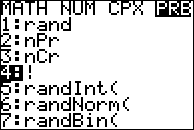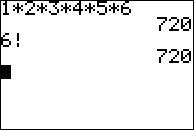Look for a " ! " button, or check your owner's manual.

• 12! = 1×2×3×4×... Oh, the heck with this. Where's my calculator...?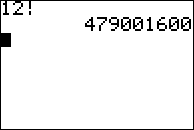12! = 479001600

When you start doing combinations, permutations, and probability, you'll be simplifying expressions that have factorials in the numerators and the denominators. For instance:

• Simplify the following:
•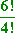I can do this in my calculator:I can also work from the definition of a factorial: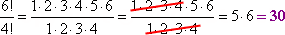In either case, 6! ÷ 4! = 30

Note how I was able to cancel off a bunch of numbers in the previous problem. This is because of how factorials are defined, and this property can simplify your work a lot.

• Simplify the following:
•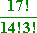Right away, I can cancel off the factors 1 through 14 that will be common to both 17! and 14!. Then I can simplify what's left to get: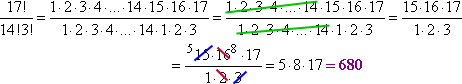Notice how I shortened what I had to write by leaving a gap (the "ellipsis", or triple-period) in the middle. This gap-and-cancel process will become handy later on (like in calculus, where you'll use this technique a lot), especially when you're dealing with expressions that your calculator can't handle. For instance:

• Simplify the following:
•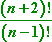My calculator can't evaluate this for me, since I'm dealing with variables rather than numbers. I'll have to simplify this by hand. To do this, I will write out the factorials, using enough of the factors to have stuff that can cancel off. Thinking back to "number" word problems, consecutive whole numbers are one unit apart, so the factors in the product (n + 2)! are of the form:

1×2×3×4×...×(n  1)×(n)×(n + 1)×(n + 2)

By going back down the list of factors as far as "n  1", I have created a list of factors that can cancel out: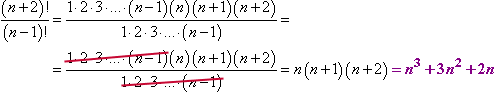Make note of the way I handled that cancellation. I expanded the factorial expressions enough that I could see where I could cancel off duplicate factors. Even though I had no idea what n might be, I could still cancel. File this technique away in your brain, because even if you don't need it now, you will almost certainly need it later.

For information on finding the number of zeroes at the end of a factorial (like "How many zeroes are at the end of 23! after you multiply it out?"), look at this note.